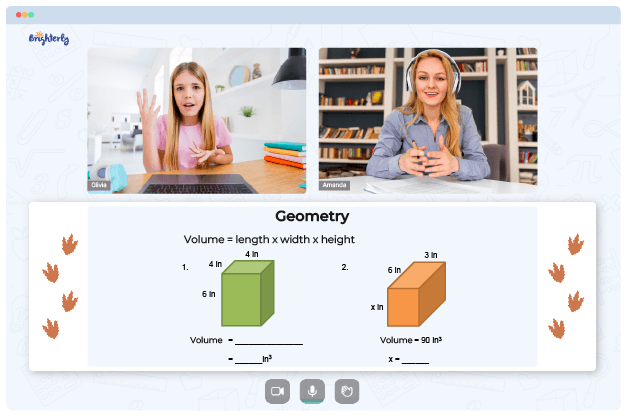# Angles In A Triangle Worksheets

Triangles have three sides and three points of intersection, with the sum of all three angles being 180°. Kids can research polygons like triangles by defining and analyzing the angles of shapes. Students can use sides of a triangle (scalene, isosceles, or equilateral) during classification. So, here is everything you should know about angles in a triangle worksheet for kids.### Angles In A Triangle Worksheet PDF

Angles In A Triangle Worksheet### Angles In A Triangle Worksheet PDF

Triangle Angle Sum Worksheet### Angles In A Triangle Worksheet PDF### Angles In A Triangle Worksheet PDF

Angles Of Triangles Worksheets

Brighterly’s tutors help youngsters with studying elementary math online. Triangles and angles are difficult for kids, so tutors employ triangle angle sum worksheets to help students learn the topic more thoroughly.

Triangles have two types of angles — interior and exterior. Interior angles are triangles’ insides, whereas exterior angles are their sides (skeleton). Using worksheets on interior and exterior angles of triangles, tutors can teach pupils the topic in an engaging way.

Math for Kids

Is Your Child Struggling With Math?
1:1 Online Math Tutoring## How to Learn Excitingly Using Angles in a Triangle Worksheets

Children quickly learn that polygons with three sides are triangles because worksheet creators simplify geometric grammar and terms. Children can use angles of a triangle worksheets to learn elementary facts, like the one that a triangle’s angles’ total is 180 degrees. Summing a triangle’s three angles also equals 180 degrees. Geometry requires knowing the essentials.

A triangle angle sum worksheet helps students comprehend triangle forms and sizes. Your kid can use a triangle angle sum worksheet with answers to crosscheck the solutions. This part of any angles of triangles worksheet is handy if you’re not constantly available to help your kid.

### More Angles Worksheets

Struggling with Geometry?• Does your child need extra help with understanding geometry lessons?
• Start studying with an online tutor.

Is your child finding it hard to grasp the geometry? An online tutor could be of assistance.